# How to Display User-Defined Text in All Blank Cells in Excel

Sometimes there are some blank cells exist in created table, if we want to mark them with user-defined text to make them easier to be found out, how can we do? Actually, there are several ways to implement this. In this article, we will introduce you two tricks to display user defined text in blank cells, the first one is applying IF function, the second one is applying Go to special function in excel.

Precondition:

Prepare a table record decrease and increase status for amount. If amount is increased, no comment records. So, if we want to enter ‘Increase’ in all blank cell, we can follow below two methods to display ‘Increase’.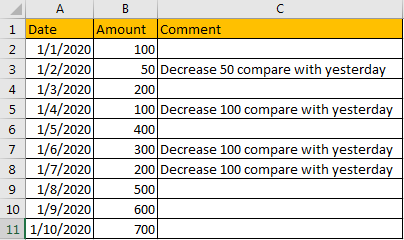## Method 1: Display User-Defined Text in All Blank Cells by IF function

As we all know, if logic is True, IF function will return value of True, so we can set user-defined text as true value, and use IF function here to return user-defined text.

Step 1: In D2, enter the formula =IF(C2=””,”Increase”,C2), then click Enter.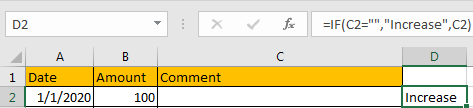Verify that Increase displays as we expect.

Step 2: Drag the fill handle down. Verify that if Cn cell is blank, then Dn cell displays Increase, otherwise text in Cn will be copied to Dn as well.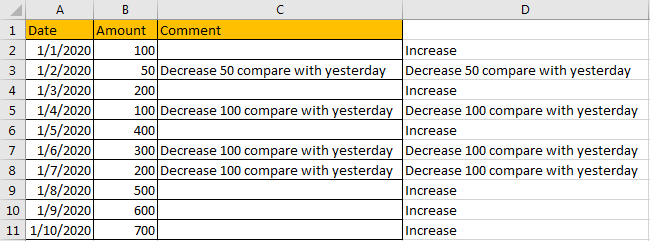Step 3: Copy D2:D11 to C2:C11. Then delete column D. Then all blank cells in table are filled with user-defined ‘Increase’ properly.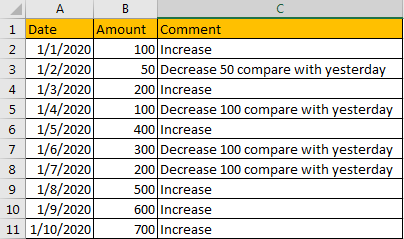## Method 2: Display User-Defined Text in All Blank Cells by Go to Special Function

Step 1: Select the range contains blank cells.

x
How to Create Dynamic Interactive Charts in Excel

Step 2: Click Home in ribbon, then click Find & Select in Editing group.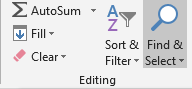Step 3: Select Go To Special in Find & Select menu.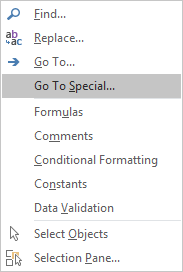Step 4: In Go To Special screen, check on Blanks option. Then click OK.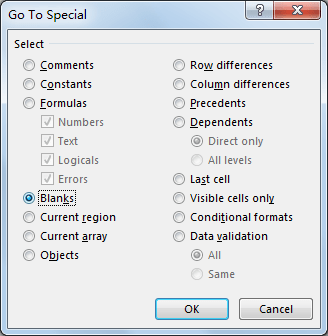Step 5: After above operating, all blank cells are selected. Type ‘Increase’ directly and press Ctrl + Enter.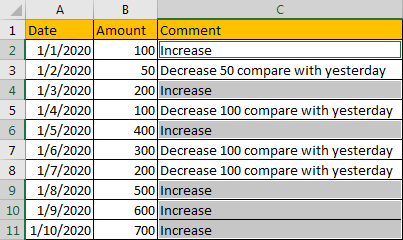Verify that all blank cells are entered with ‘Increase’.

### Related Functions

• Excel IF function
The Excel IF function perform a logical test to return one value if the condition is TRUE and return another value if the condition is FALSE. The IF function is a build-in function in Microsoft Excel and it is categorized as a Logical Function.The syntax of the IF function is as below:= IF (condition, [true_value], [false_value])….

Related Posts

If Cell is This Value or That Value

IF function is frequently used in Excel worksheet to return you expect “true value” or “false value” based on the result of logical test. If you want to see if a cell is A or B, and if one of ...

If Value is Greater Than A Certain Value

IF function is frequently used in Excel worksheet to return you expect “true value” or “false value” based on the logical test result. If you want to see if a value in one cell is greater than a specific value, ...

If Cell is Not Blank

IF function is frequently used in Excel worksheet to return you expect “true value” or “false value” based on the result of created logical test. If you want to see if a cell is blank or not, and leave some ...

If Cell is Blank

IF function is frequently used in Excel worksheet to return you expect “true value” or “false value” based on the result of created logical test. If you want to see if a cell is blank or not, and leave some ...

If Cell Equals Certain Text String

IF function is frequently used in Excel worksheet to return you expect “true value” or “false value” based on the result of created logical test. If you want to see if cell equals a certain text string like “Win”, you ...

If Cell Contains Either Text1 or Text2

IF function is frequently used in Excel worksheet to return “true value” or “false value” based on the logical test result. If you want to see if cell contains certain substring1 like “abc” or substring2 like “def”, and returns true ...

If Cell Contains Certain Text OR Equals Certain Text

IF cell equals certain text IF function is frequently used in Excel worksheet to return “true value” or “false value” based on the logical test result. If you want to test values to see if they equal certain text like ...

Fix #N/A Error For VLOOKUP From Another Sheet

This post will show you how to fix the #N/A error why it occurs when you extract values from another sheet using VLOOKUP function in Excel 2016,2013,2010 or other Excel versions. How can you correct a #N/A error in VLOOKUP ...

How to Average Only Positive or Negative Numbers of a Range

Suppose both positive numbers and negative numbers exist in a table. If we want to know the average of only positive numbers in this table, we can create a formula to get average of all positive numbers with all negative ...

VLOOKUP with Two Lookup Tables

VLOOKUP is one of the key functions among all lookup & reference functions in Excel. Today we will show you the application of VLOOKUP function when there are two lookup tables. EXAMPLE Table1 and table2 record the rates of Y2020 ...

Sidebar